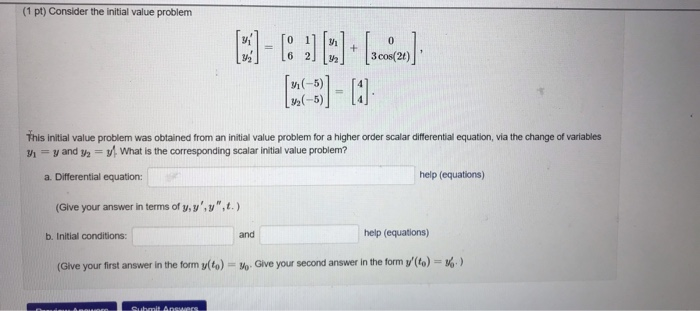# Solved: (1 Pt) Consider The Initial Value Problem 0 11 6 2 3 Cos( N(-5)] -5) This Initial Value Problem Was Obtained From An Initial Value Problem For A Higher Order Scalar Differential Equation, Via

By |(1 pt) Consider the initial value problem 0 11 6 2 3 cos( n(-5)] -5) This initial value problem was obtained from an initial value problem for a higher order scalar differential equation, via the change of variables 1 y and 3h= What is the corresponding scalar initial value problem? help (equations) a. Differential equation: (Give your answer in terms of y, y',y",t.) help (equations) b. Initial conditions: and (Give your first answer in the form y(to)Mo Give your second answer in the form y'(te) = . ) Submit Answers.